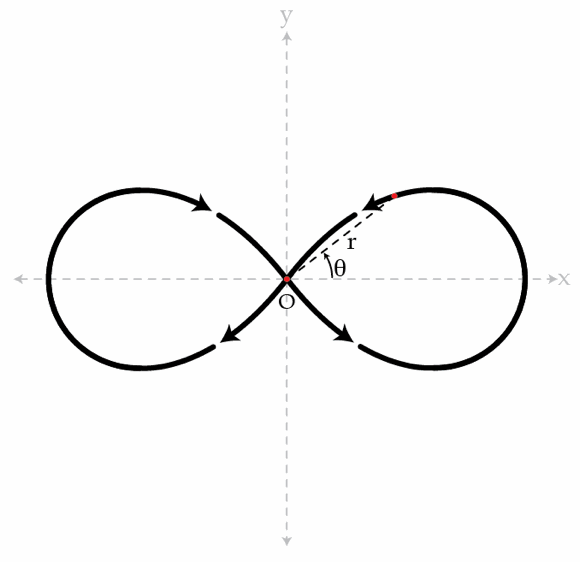# An interesting trajectory

A particle describes a path (see the figure below) which in polar coordinates is given by the equation $r^{2}= R^{2}\cos(2\theta).$ It turns out that such trajectory is possible when the particle moves under the influence of a central force of the form $F(r)=\frac{C}{r^{n}}$ where $C$ is a constant, $r$ is the distance to the origin O and $n$ is an integer. Determine $n$.Note that a central force is always directed toward a fixed point (point O in this case).

Details and assumptions

• Consider only one half of the diagram, i.e. the particle travels around the left lobe or the right lobe, but not both.
×